Home > A2C > Chapter 8 > Lesson 8.2.1 > Problem8-116

8-116.
1. Imagine the graph y = sin(x) shifted up one unit. 8-116 HW eTool (Desmos). Homework Help ✎

1. Sketch what it would look like.

2. What do you have to change in the equation y = sin x to move the graph up one unit? Write the new equation.

3. What are the intercepts of your new equation? Label them with their coordinates on the graph.

4. When you listed intercepts in part (c), did you list more than one x-intercept? Should you have?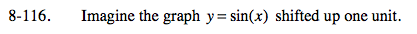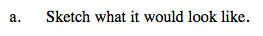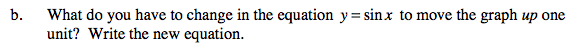In the general equation y = sin (xh) + k, which parameter moves this graph vertically?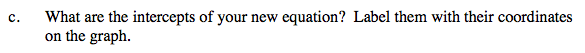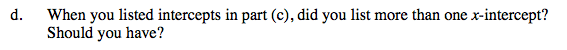See part (c). At what interval are the x-intercepts located?

Use the eTool below to help answer the parts of the problem.
Click the link at right for the full version of the eTool: A2C 8-116 HW eTool